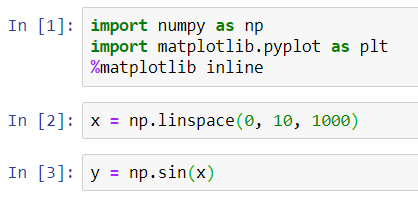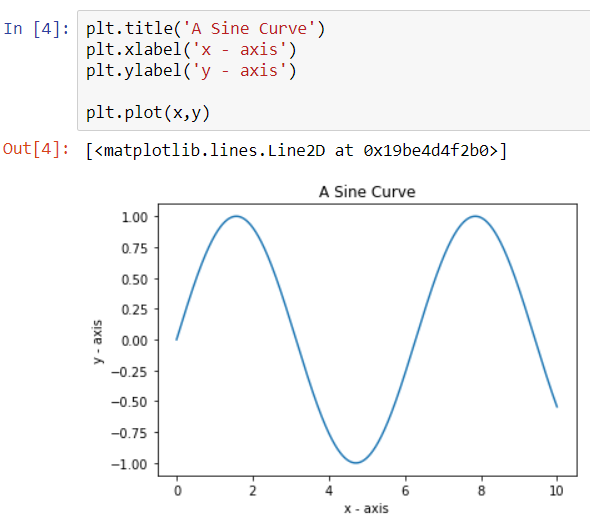When we create a plot in Matplotlib, we can add some general info by labeling it. We can give our plot a title, we can label our axis, etc.

Let us begin by importing the Matplotlib library and creating a small dataset for which we will plot a sinusoidal curve.#### Adding a Title to the plot & Naming the coordinate axis

To give our plot a title, we use the function pyplot.title(String) and to give labels to the x-axis and y-axis, we use the functions pyplot.xlabel(String) and pyplot.ylabel(String) respectively.